# C 510: Quantum Computing (20 pts)

## What you need

• Any computer with Python 3

## Purpose

To practice using a simulated quantum computer for very basic operations.

## Installing ProjectQ

Execute these commands:
```python3 -m pip install --upgrade pip python3 -m pip install --user projectq ```

## A Qubit in State |0>

Normal computers use bits, which are always 0 or 1. Quantum computers use qubits, which are more complex than bits. However, qubits can be used to encode bits, as we will do here.

In a text editor, create a file named q1.py containing this code:

```from projectq.ops import Measure from projectq import MainEngine quantum_engine = MainEngine() print("Default Qubit in state |0>", end=" ") for i in range(10): qubit = quantum_engine.allocate_qubit() Measure | qubit quantum_engine.flush() print(int(qubit), end=" ") print() ```
Run the program.

A newly created Qubit is in the state `|0>`, which means that measuring the state of this Qubit always results in 0, as shown below.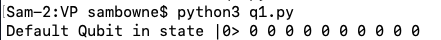## Flipping a Qubit with an X Gate

In a text editor, create a file named q2.py containing this code:
```from projectq.ops import Measure, X from projectq import MainEngine quantum_engine = MainEngine() print("Qubit in state |0>", end=" ") for i in range(10): qubit = quantum_engine.allocate_qubit() Measure | qubit quantum_engine.flush() print(int(qubit), end=" ") print() print("Qubit in state |1>", end=" ") for i in range(10): qubit = quantum_engine.allocate_qubit() X | qubit Measure | qubit quantum_engine.flush() print(int(qubit), end=" ") print() ```
Run the program.

the X gate flips the Qubit to the state `|1>`. Measuring that Qubit always results in 1, as shown below.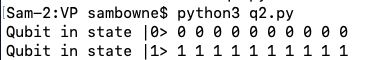## Superposition State

So far we've used Qubits to to the same thing normal bits do. To see the unique properties of Qubits, we need to use "superposition" states that are mixtures of `|0>` and `|1>`.

The Hadamard gate moves the Qubit into a superposition state containing equal amounts of `|0>` and `|1>`.

In a text editor, create a file named q3.py containing this code:

```from projectq.ops import Measure, H from projectq import MainEngine quantum_engine = MainEngine() print("Qubit in state |0>", end=" ") for i in range(10): qubit = quantum_engine.allocate_qubit() Measure | qubit quantum_engine.flush() print(int(qubit), end=" ") print() print("Superposition State: ", end=" ") for i in range(10): qubit = quantum_engine.allocate_qubit() H | qubit Measure | qubit quantum_engine.flush() print(int(qubit), end=" ") print() ```
Run the program several times. The superposition state has a 50% chance of being 0 when measured, and a 50% chance of being 1, as shown below.

This is "quantum uncertainty" and it is a fundamental aspect of reality that changed everything about physics. It bothered Einstein, who said "God does not play dice." However, as far as we can tell, Einstein was wrong and this uncertainty is a real property of quantum objects.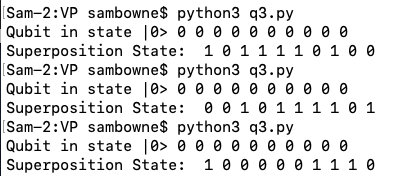## Entanglement

It is possible to "entangle" two Qubits, so that they must both have the same value when measured. This is an important feature of Qubits that does not happen with regular bits, and it makes some calculations far faster on quantum computers.

To entangle Qubits, we use the CNOT operator.

In a text editor, create a file named q4.py containing this code:

```from projectq.ops import Measure, H, CNOT from projectq import MainEngine eng = MainEngine() for i in range(10): qubit1 = eng.allocate_qubit() qubit2 = eng.allocate_qubit() H | qubit1 CNOT | (qubit1, qubit2) Measure | qubit1 Measure | qubit2 eng.flush() print("(", int(qubit1), int(qubit2), ")", end=" ") print() ```
Run the program several times. The Qubits are sometimes 0, sometimes 1, but they are always equal, as shown below.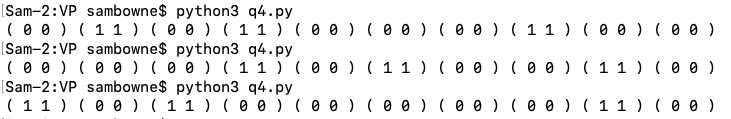## Pretty Circuit Diagrams

Install LaTeX. On a Mac, execute the command below. For other operating systems, see this page.
```brew install --cask mactex ```
The installation took 15 minutes on my system.

In a text editor, create a file named q5.py containing this code:

```from projectq.ops import Measure, H, CNOT from projectq import MainEngine from projectq.backends import CircuitDrawer drawing_engine = CircuitDrawer() eng = MainEngine(drawing_engine) qubit1 = eng.allocate_qubit() qubit2 = eng.allocate_qubit() H | qubit1 CNOT | (qubit1, qubit2) eng.flush() print(drawing_engine.get_latex()) ```
Execute these commands to write a LaTeX file and convert it to a PDF file:
```python3 q5.py > q5.tex pdflatex q5.tex ```
When I did this on 12-28-20, "pdflatex" failed with this error: "! LaTeX Error: File `standalone.cls' not found."

I used this online conversion site instead, which worked:

https://www.freefileconvert.com/

Browse to q5.pdf and double-click it. You see a pretty diagram of your quantum circuit, as shown below.

The two Qubits start in their default states of `|0>` .

One of them passes through a Hadamard gate, and then they are entangled, denoted by the the symbol.# C 510.1: Three Qubits (10 pts)

Program the quantum circuit shown below.

Note that the symbol on a single line denotes an X gate.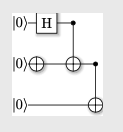Run that circuit a few times, printing the three bits. The answers vary.

Find the answer containing the largest number of ones, and concatenate the bits, like this:

```001 ```
That's the flag.

# C 510.2: Five Qubits (10 pts)

Program the quantum circuit shown below.

Note that the symbol on a single line denotes an X gate.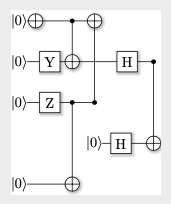Run that circuit a few times, printing the five bits. The answers vary.

Interpret each sequence of five bits into a binary number, and convert it to decimal.

The highest possible value is the flag.

For example, if the bits were

```11111 ```
the flag would be 31.

## Sources

IBM Q Beginner's Guide
Quantum Computer Programming Introduction
Tutorial: Getting started with Quantum Computing in Python
Quantum teleportation
ProjectQ Docs Examples

Posted 7-12-2020 by Sam Bowne
PDF instructions updated 12-28-20